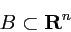### The Schrödinger equation

• Time evolution of the wave function ψ(x,t):
• in suitable units given by
•• with the Hamilton operator
•• where• Interpretation of ψ:
• normed solutions in L2(Rn) describe a particle, i.e.
•• probability p(B) to find the particle in region•• Numerical solution:
• finite differences in x and t
• based on algorithm by DeRaedt (4th order in dx and dt)
• "perfectly matched layer" → no boundary reflections